# Invariants for a State Machine

#### (A new question of the week)

Although we focus in this blog on questions at early college level and below, we do get questions at higher levels. This one deals with finding an invariant for a finite state machine, with possible movements of a robot as the example.

## First problem (relatively easy)

The question came to us last December, from Akinyemi:

The question is on discrete mathematics (state machine: invariant principle)

Have been trying solving it but wasn’t able to get the solution.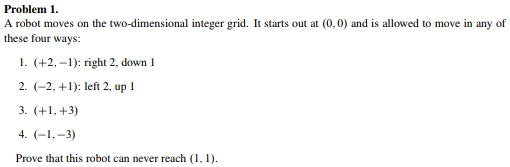Here are the four moves: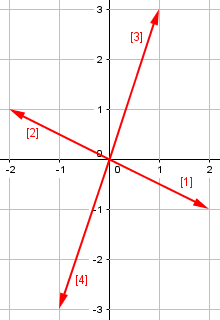Doctor Jacques replied, asking for contextual information and making an initial suggestion:

Hi,

It would help us to give you the most useful answer it you told us what you have been studying on this subject, and similar problems you have been solving, in particular about finding invariants.

I can give you some ideas to get you started. Note first that the moves (1) and (2) on the one hand, and (3) and (4) on the other hand, are inverses of each other. This means that you can use only the moves (1) and (3), for example, if you allow negative moves.

More precisely, you can describe any sequence of moves as m(2, -1) + n(1, 3), where m and n are (possibly negative) integers.

The key idea here is that move  is the opposite of move , and move  is the opposite of move ; that means the robot can go back and forth in either of the two directions, which amounts to positive or negative multiples. This is not always true, and will not be in our second problem.

You can then set this equal to (1, 1); this will give you a linear system of two equations (corresponding to the x and y coordinates):

2m + n = 1
m + 3n = 1

If you solve that system, you will find that m and n are not integers.

Because these variables are not integers, there is no whole number of moves by which the robot could reach (1, 1). But this is not what the author of the problem asked for:

This answers the question. However, this does not use the concept of invariant; I could give you an invariant right away, but it would look like black magic; it would not tell you how to find the invariant. That is why I asked you how you were told to answer that type of question.

Here is a hint: try first to solve the system of equations above: the denominator in the solution will tell you what kind of invariant you need.

Please feel free to write back if you need further help, and don’t forget to tell us what you learned about this type of problem.

Sometimes finding an invariant for a new problem can be magic. But there’s no sense making it look like that when it isn’t …

### Context

Akinyemi responded with an example he was using (which I have since found is available at MIT OpenCourseWare):

Thanks for your quick response towards my question, I really appreciate.

I tried getting your method but not yet comfortable with it.

Regards what have been studying on this work are the pictures attached below.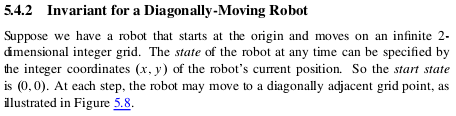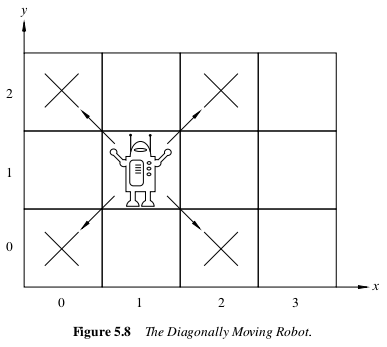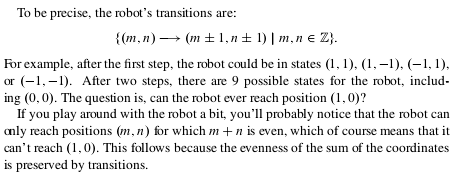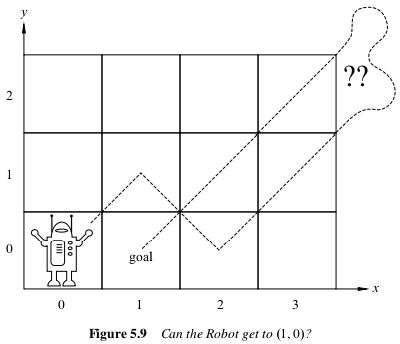Kindly go through it and help with any further assistance.

(He included more from the book showing details of a proof of the invariant, which I am omitting.)

In this example, the four allowed moves are simpler: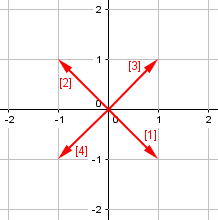The invariant they use (that is, a property of the location of the robot that must always be true because it is preserved by each legal move) is that the sum of the coordinates is even. Whatever location you start at, changing both x and y by 1 will either leave the sum unchanged, or increase it or decrease it by 2; so if that sum started even, it will stay even.

### Finding an invariant, two ways

Doctor Jacques replied, first showing how to use his hint (solving the system of equations) to find the invariant:

Hi again,

We want to find a property of the coordinates that is preserved by the moves of the robot.

If you try to solve the system of equations above, you will find m = 2/7, n = 3/7. In the examples you showed me, the condition to be preserved was divisibility by 2. In this example, the denominator 7 suggests that we must find an expression that must be divisible by 7 (to get integral values for m and n).

A little trial and error will show that, for example, I1 = 3x – y is such an expression. Indeed, I1 is divisible by 7 for the starting position (0, 0) and is divisible by 7 for the two moves (2, -1) and (1, 3). You can then use the same induction argument as in your notes. As I1 is not divisible by 7 at the position (1, 1), that position is not reachable.

Adding 2 to x and -1 to y increases I1 by $$3(2) – (-1) = 7$$, retaining divisibility by 7; and similarly, adding 1 to x and 3 to y increases I1 by $$3(1) – (3) = 0$$.

Trial and error is not un-mathematical, as many people imagine; but something else would be desirable.

A more systematic way to find the invariant would be to solve the system:

2m + n = x
m + 3n = y

as a function of x and y. This gives m = (3x – y)/7 and n = (x + 2y)/7. The solution will be integral if and only if I1 = (3x – y) and I2 = (x + 2y) are divisible by 7.

Iis the invariant we found before; I2 is another invariant. Note, however, that you only need one of these, since 2I1 + I2 = 7x is always divisible by 7.

This system of equations represents the vector equation $$m\left\langle 2,-1 \right\rangle + n\left\langle 1,3\right\rangle = \left\langle x,y \right\rangle$$; that is, we are now finding how to get to any point, not just $$(1, 1)$$. By generalizing, we can more easily see patterns! The conclusion is that any point $$(x, y)$$ is accessible if $$3x-y$$ and $$x+2y$$ are both multiples of 7; but it turns out that if one is, the other is, which was shown by eliminating y to see that $$I_2$$ differs from $$2I_1$$ by a multiple of 7.

### Five clarifications

Akinyemi needed a little more help (which will be good for others who read this!):

I thank you for your kind gesture towards this my problem. You have really helped a soul sir!

Having gone through the solutions, I still find it difficult to understand the whole solution and I have come up with the following questions so as to understand clearly sir!

1. Why describing sequence of move as m(2, -1) + n(1, 3)?
2. Why setting it to (1, 1) and not other value?
3. How do we get I1 = 3x – y?
4. How do we know I1 is divisible by 7 for the starting point (0, 0) and divisible by 7 for the two moves (2, -1) and (1, 3)?
5. Why is I1 not divisible by 7 at the position (1, 1)?

Also, in the systematic way, how do we get the solution part of “iff” I1 = (3x-y) and I2 = (x+2y) are divisible by 7?

I’m really sorry for asking all these questions, this topic is strange to me and I need to understand so as to solve other problems myself.

Looking forward to hear from you.

Doctor Jacques took each question in turn:

Hi Akinyemi,

Question 1 [Why m(2, -1) + n(1, 3)?]

You can see a sequence of moves as an addition of vectors. You have two basis vectors u = (u1, u2) = (2,-1) and v = (v1, v2) = (1, 3), which correspond to moves (1) and (3) in the problem (moves (2) and (4) are –u and –v).

You add vectors by adding the corresponding components. This operation is commutative: the end result does not depend on the order of the moves. We can use m as the number of times you execute move (1) (or move (2) if m < 0), and n as the number of times you execute move (3) (or (4) if n < 0).

The final position (x, y) is therefore (mu1 + nv1mu2 + nv2) = (2m + n, –m + 3n), which we can write as mu + nv = m(2,-1) + n(1,3). Equating corresponding components gives the system of equations in my last answer:

2m + n = x
m + 3n = y (*)

This system has a unique solution (m, n). A position is reachable if and only if m and n are integers (these are numbers of moves; partial moves are not allowed).

To put it another way, the final x coordinate results from adding 2, m times (move 1), and adding 1, n times (move 3). The final y coordinate comes from subtracting 1, m times, and adding 3, n times.

Question 2 [Why (1, 1)?]

I used (x, y) = (1, 1) because that is what the problem asks for. If you solve the system (*) with x = y = 1, you will find that the solution m = 2/7, n = 3/7 does not consist of integers. As you cannot execute 2/7 of a move, the position is not reachable. This is sufficient if you only want to prove that result, but apparently, you are supposed to find an invariant to prove that result, and this calls for a more general solution, which is explained in the next section.

In the method using the invariant, (1, 1) is used in applying the invariant to answer the question; in the first method, it was used from the start.

Question 3 [How I1 = 3x – y?]

If we solve the system (*) with x and y as parameters (you know how to do that, don’t you?), we find the general solution:

m = (3x – y)/7
n = (x + 2y)/7

A position is reachable if and only if m and n are integers. This means that (3x – y) and (x + 2y) must be divisible by 7. For convenience, we call these expressions I1 and I2. As these expressions depend on x and y, it might be a good idea to call them I1(x, y) and I2(x, y) to emphasize the point. To summarize, the position (x, y) is reachable from (0, 0) iff I1(x, y) = (3x – y) and I2 (x, y) = (x + 2y) are both divisible by 7.

As I said, you only need to check one of the conditions, since 2I1 + I2 = 7x is always divisible by 7: if one of them is divisible by 7, so is the other.

Let’s carry out the solution of the system:

We want to solve $$\begin{matrix} 2m + n = x\\-m + 3n = y\end{matrix}$$

To eliminate n, we can multiply the first equation by -3 and add; we get $$\frac{\begin{matrix} -6m – 3n = -3x\\-m + 3n = y\;\;\;\end{matrix}}{-7m\;\;=-3x+y}\Rightarrow m = \frac{3x-y}{7}$$

To eliminate m, we can multiply the second equation by 2 and add; we get $$\frac{\begin{matrix} 2m + n = x\\-2m + 6n = 2y\;\;\;\end{matrix}}{7n\;\;=x+2y}\Rightarrow n = \frac{x+2y}{7}$$

Question 4 [How do we know I1 is divisible by 7?]

You just have to substitute x and y in I1(x, y) = (3x – y). This gives I1(0, 0) = 0, I1(2, -1) = 7, and I1(1, 3) = 0, and these are all divisible by 7.

Question 5 [Why is I1 not divisible by 7 at (1, 1)?]

We have I1(1, 1) = 2, and this is not divisible by 7; therefore this point is not reachable.

The idea in question 4, of course, is that I1 must be divisible by 7 in order to have a solution (whole moves); while in question 5, it is that I1 is not  be divisible by 7 when calculated for the point (1, 1), which shows that there is no solution in this case. These are two sides of the same coin, and it is likely that Akinyemi was looking at the wrong sides!

### Modular arithmetic

I would like to add a few remarks.

If you are familiar with modular arithmetic, you can say that I1(x, y) mod 7 does not change during a sequence of moves, since each move adds a multiple of 7 to it. This corresponds more closely to the meaning of an invariant expression: I1(x, y) mod 7 does not change. Otherwise, we have an invariant condition (instead of expression): I1 must be divisible by 7. The modular formalism has the advantage that you can use it easily if you have another starting point instead of (0, 0).

Any knowledge of number theory techniques can make this topic easier to handle.

### Making it visual

Sometimes, the invariant has an intuitive meaning. In the example you showed me with the robot moving diagonally, you can imagine the robot moving on the squares of a chessboard. If the squares with (x + y) even are colored black and the others white, the condition expresses the fact that the robot stays on squares of the same color (like a Bishop in chess). The invariant is the color of the square in this case.

Here is that example, superimposed on a chessboard: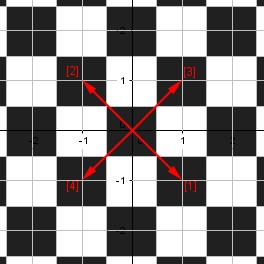The color remains black after any move.

There is also a nice geometric interpretation of your problem. You can construct a parallelogram on the vectors u and v representing the moves, and tile the whole plane with copies of that parallelogram.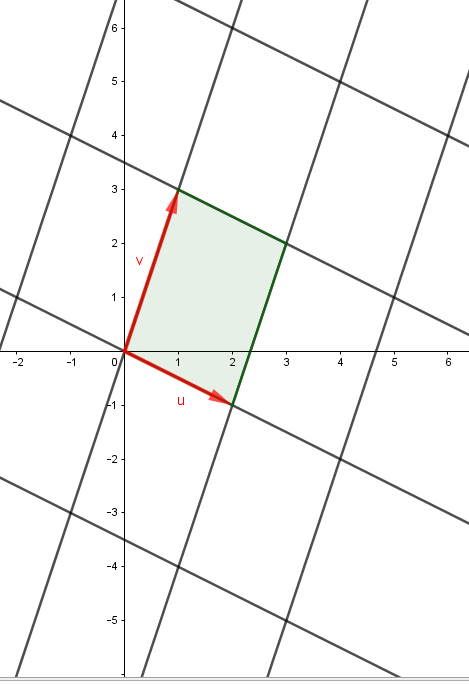Because you can only move along the sides of these parallelograms, the only positions reachable from the origin are the vertices of the parallelograms. As the point (1, 1) is inside a parallelogram, it is not reachable. This allows you to answer the question without any calculation.

The key here is to see clearly that the grid constrains all possible motions in this system; without that, the problem can seem impossible, but with it, it is obvious!

## A harder problem

Akinyemi responded with thanks and a further challenge:

Greetings Dr,

Kudos to you! You are a great teacher. With your explanation, I was able to attempt a question on my own. Attached is the problem I solved but needs your observation whether I’m right or wrong.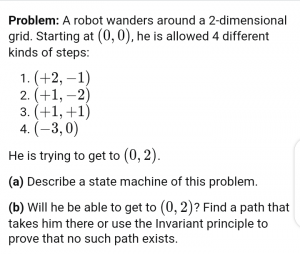Solving the problem, I used moves (3) & (4) which leads to the system of equations:

M = y and n = (y – x)/3.

Since we can only use either of the invariant, I used y – x to check and all the conditions were satisfied except the given condition I(0, 2) which is not divisible by 3 which implies the point is not reachable.

Thanks so much Dr!

Here are the moves for this problem: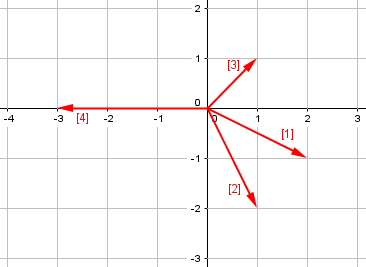Hi Akinyemi,

This problem is different from the previous one. In the previous one, we had pairs of moves that are inverse of each other, and this allowed us to use only one move for each pair, together with possibly negative coefficients.

This is not the case here. I understand that you cannot use a move like - = (-2, +1) (at least, not directly, see below for more).

This means that you must use all four moves, and only positive coefficients.

The short method is to observe that, in all four moves, the difference x – y is a multiple of 3. You noticed this for moves  and , but that is not enough: you must check it for all moves. As this is true for the starting position, this is an invariant condition. As it does not hold for (0, 2), that position is not reachable. Note that this is sufficient to reach the conclusion: it does not depend on the fact that negative moves are not allowed.

This requires some minimal guessing; you can observe that, if you solve the system of equations for all possible pairs of moves, you will find in each case that something must be divisible by 3. This suggests looking for something that is divisible by 3 in each move.

You can see this invariant, that each move changes x and y by a sum of 3, visually, by observing that the points all lie on the lines shown here, namely $$x-y = -3, 0, 3$$: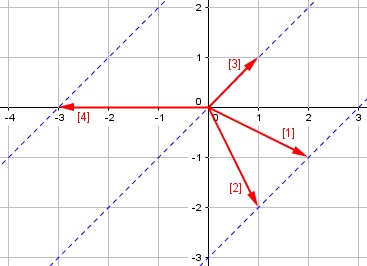Now for the long method. This may require some techniques that you are not familiar with (although this case is simpler than the general case), and I would be surprised if you were expected to do this. If, while reading this, you have no idea what I am talking about, just ignore it; as I said, I believe that the short method is all you need. Anyway, here it goes:

— Start of digression —

I said that you cannot use directly the inverse of the moves. However, in this case, it turns out that you can achieve the same result with combinations of moves. For example, move -  = (-2, +1) can be simulated as  + . Explicitly, we have the equivalences:

- = (-2, 1) = (+1, +1) + (-3, 0) =  + 
- = (-1, +2) = 2(+1, +1) + (-3, 0) = 2 + 
- = (-1, -1) = (+2, -1) + (-3, 0) =  + 
- = (+3, 0) = (+2, -1) + (+1, +1) =  + 

Note that, in each case, we use only positive coefficients, as required. At this point, a couple of remarks are in order:

• I found these equivalences by inspection (which means that, after all, this method is not better than the short method). In general, there is a systematic method for finding these equivalences if they exist, but that requires solving systems of Diophantine equations in several variables.
• In this case, we are lucky that such equivalences exist. If this is not the case, I don’t know if a general method exists; we would probably need an ad hoc method.

We have shown that we can, in fact, use all the four moves  –  with positive or negative coefficients.

We need now to reduce this set to only two moves; this will allow us to use the technique of the previous problem. The general technique is called reduction to Hermite normal form and described here. However, in this case, we already have the answer: you can generate all moves using  and , possibly with negative coefficients (we had to prove that part first). Indeed, if we change the signs of the first two equivalences, we find:

 = - – 
 = -2 – 

— End of digression —

We have therefore proved that we can use only moves  and , possibly with negative coefficients. In fact, this is what you did, but you have to prove first that it is legitimate; it is not an immediate consequence of the hypotheses.

The solution of the system of equations is m = yn = (y – x)/3, as you found.

Akinyemi was right, and yet wrong in not backing up what he did with proof.

However, it is not true that you can use either invariant in this case: that was true for the previous problem, but it is not true here. In fact, m = y only tells you that y is an integer, and that is not helpful. You must use the second invariant: (y – x) must be a multiple of 3. This is because the denominator 3 only appears in the second invariant.

I think that the conclusion of all this is that you should use the short method: observe that (y – x) is a multiple of 3 in all 4 moves. This is sufficient to prove the conclusion, and you don’t need to prove that you can use only the moves  and .

Again, “inspection” (like trial and error) is respectable!

This site uses Akismet to reduce spam. Learn how your comment data is processed.